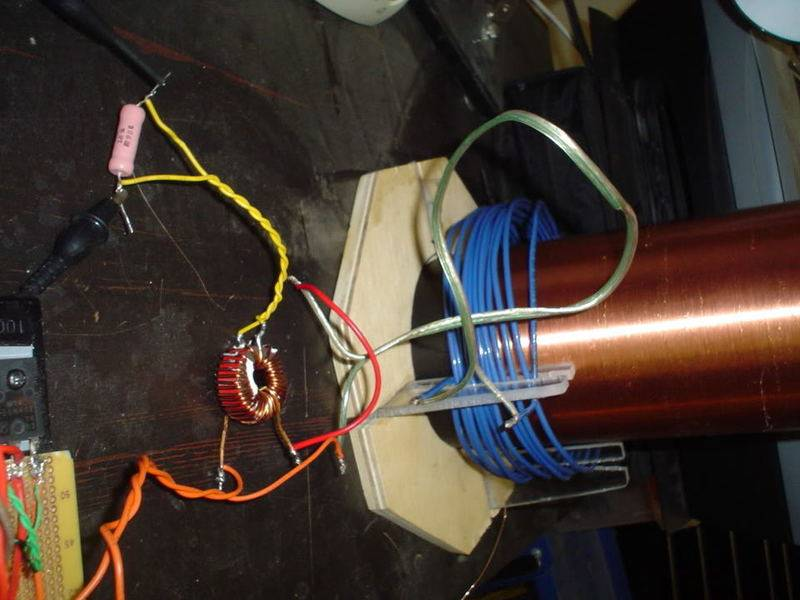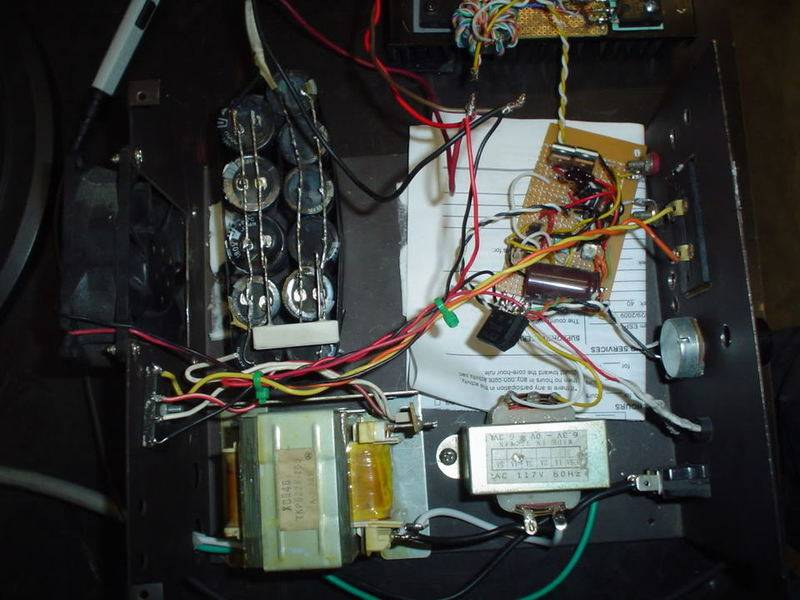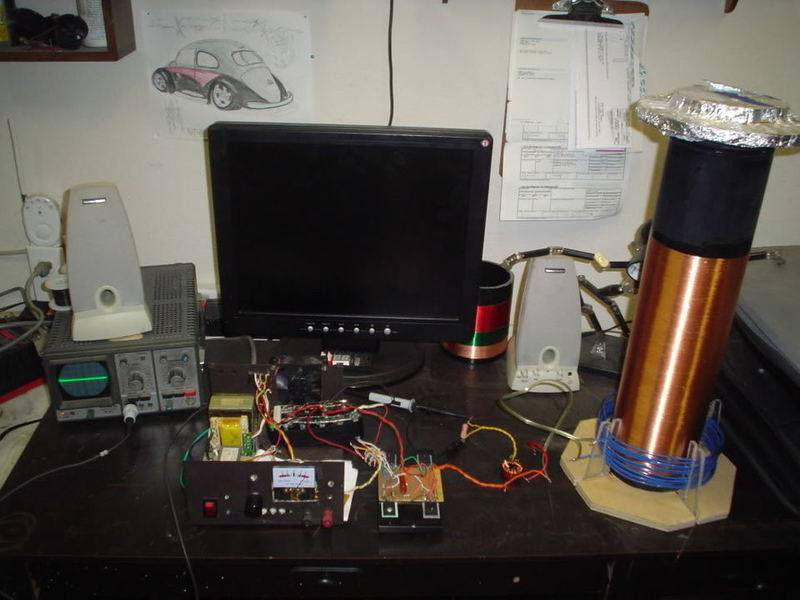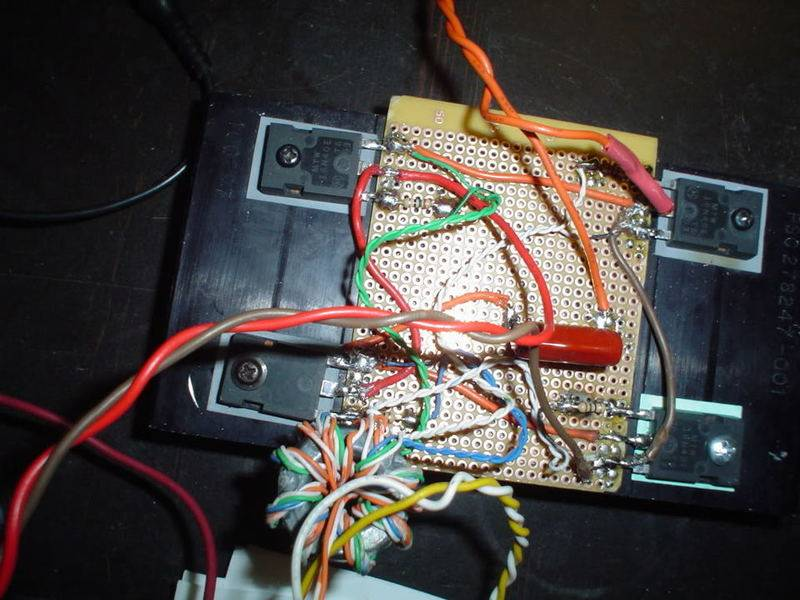# Question about RMS power, confused a little

Hi everyone, I have a question maybe one of you can help me out.
I have been working on a solid state tesla coil made with mosfets and a sg3524 PWM chip, I have got it working and am in the process of adding a current limiter to it (Mosfets can get expensive). The current limiter works with a current transformer on the output from the H bridge and has a 2:33 turns ratio with a 1.5 ohm resistor. By measuring the voltage across the resistor, I can calculate current using ohm's law. That part is all fine and good. My problem is that in my experience I have always worked with a sine wave current AND voltage, so finding rms watts was a simple matter of 1.414. With this setup however, I have a squarewave voltage waveform and a sinewave current waveform, to me it doesn't seem like the same equation would apply, thoughts?

dlgoff
Gold Member
I did a little searching and found this:
Abstract: The rms (root-mean-square) value of various waveforms is determined without the use of calculus, which should be of benefit to the Technology student. These waveforms include the half-wave rectified sinusoid, the quarter-wave rectified sinusoid, a waveform consisting of a dc voltage added to a sinusoid, the sum of two sinusoids, which may be periodic or aperiodic, a triangular waveform, and a pulse train. Furthermore, in order to compute the rms value, the mean of the square of a voltage must be determined over an observation time. A discussion of the importance of using the correct observation time is also provided.
http://technologyinterface.nmsu.edu/Fall07/" [Broken]

Last edited by a moderator:
vk6kro
With a true square wave and a resistive load, you can work out the RMS power by inspection.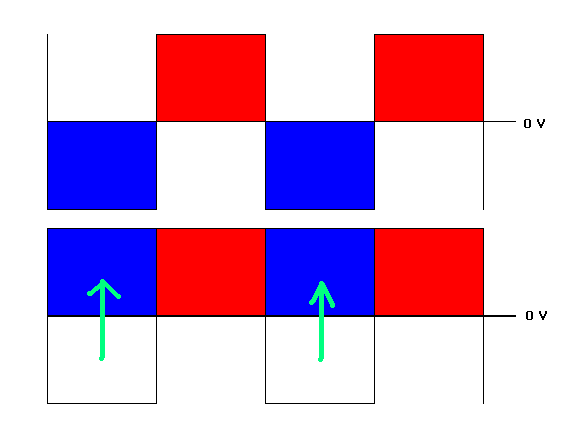The power is just the peak voltage times the peak current. See diagram. The voltage and current can be redrawn as a DC voltage and current.

If the voltage is a DC pulse, ie with no negative (or no positive ) component, The power is the peak voltage times the peak current times the duty cycle.

However, if the load is not resistive, simple solutions become impossible and you have to start measuring when current flows at the same time as voltage is applied.

You also have to consider whether you are obsessive enough to care. :)

Thank you dlgoff, I read the article and it helped a bit, It turns out I was just over-thinking it. When Ive calculated Rms power in the past, like I said, it was with a sine wave voltage, but I forgot that when I've done so, It has been using the rms voltage already. So this circuit having a square wave voltage, with a near 100% duty cycle, the Rms voltage would be the same as the peak voltage anyways, so I am fairly confident the formula still applies.

Ok, so here is what I have so far, perhaps one of you can double check for me and make sure I am doing this right. (including the current transformer part)
voltage is a squarewave of +/- 70V, Current is 10.3A Pk, found by scoping current transformer output (2:33 turn, 1.5 ohm resistor and 1v measured across resistor) in a sinewave (coil operated in resonance so L and C values cancel eachother out). So 10.3A/1.414=7.28ARms, 7.28ARms X 70VPk = 509.6 watts rms and 721 watts peak. Does all this seem right?

vk6kro
I think you would really need to post at least a block diagram of where you are measuring these voltages etc.

If you are applying a square wave voltage and getting a sinewave current, then the load is not resistive.

Ive added some photos for you, the two orange wires are the full bridge ac output @172Khz, one goes to the TC primary, the other goes to the two turns on the current transformer (looks like a filter torrid) the secondary has another 33 turn winding on it, which is connected to the two smaller yellow wires, connected to the pink-ish 1.5ohm, 5W, Non inductive resistor with the scope probe connected. Scope is set to 1V/Div and .2uS/Div. and I am getting a sinewave 1VPk out of the current transformer. Voltage is simply the DC input voltage to the bridge from the large transformer in the back of the box.